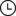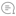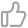# Empyrical 超简单教你一行代码计算风险指标Python实用宝典 |1032022-05-29 07:32000

Empyrical 是一个知名的金融风险指标库。它能够用于计算年平均回报、最大回撤、Alpha值、Beta值、卡尔马率、Omega率、夏普率等。它还被用于zipline和pyfolio，是Quantopian开发的三件套之一。

1.准备

(可选1) 如果你用Python的目的是数据分析，可以直接安装Anaconda：Python数据分析与挖掘好帮手—Anaconda，它内置了Python和pip.

(可选2) 此外，推荐大家用VSCode编辑器，它有许多的优点：Python 编程的最好搭档—VSCode 详细指南

1. Windows 环境 打开 Cmd (开始-运行-CMD)。
2. MacOS 环境 打开 Terminal (command+空格输入Terminal)。
3. 如果你用的是 VSCode编辑器 或 Pycharm，可以直接使用界面下方的Terminal.

``pip install empyrical``

2. Empyrical 基本使用

``import numpy as npfrom empyrical import max_drawdownreturns = np.array([.01, .02, .03, -.4, -.06, -.02])# 计算最大回撤max_drawdown(returns)# 结果：-0.4472800000000001``

``import numpy as npfrom empyrical import alpha_betareturns = np.array([.01, .02, .03, -.4, -.06, -.02])benchmark_returns = np.array([.02, .02, .03, -.35, -.05, -.01])# 计算alpha和Beta值alpha, beta = alpha_beta(returns, benchmark_returns)print(alpha, beta)# 结果：-0.7960672549836803 1.1243025418474892``

``import numpy as npfrom empyrical import sharpe_ratioreturns = np.array([.01, .02, .03, -.4, -.06, -.02])# 计算夏普率sr = sharpe_ratio(returns, risk_free=0, period='daily', annualization=None)print(sr)# 结果：-6.7377339531573535``

3.更多的指标

Empyrical 能提供使用的指标非常多，这里就不一一介绍了，基本上用法都和夏普率的计算方法差不多，这里介绍他们的方法和参数。

3.1 omega_ratio

``empyrical.omega_ratio(returns, risk_free=0.0, required_return=0.0, annualization=252)``

3.2 calmar_ratio

``empyrical.calmar_ratio(returns, period='daily', annualization=None)``

3.3 sortino_ratio

``empyrical.sortino_ratio(returns, required_return=0, period='daily', annualization=None, _downside_risk=None)``

https://github.com/quantopian/empyrical/blob/master/empyrical/stats.py

Python实用宝典 (pythondict.com)00

暂无评论~~
Ctrl+Enter